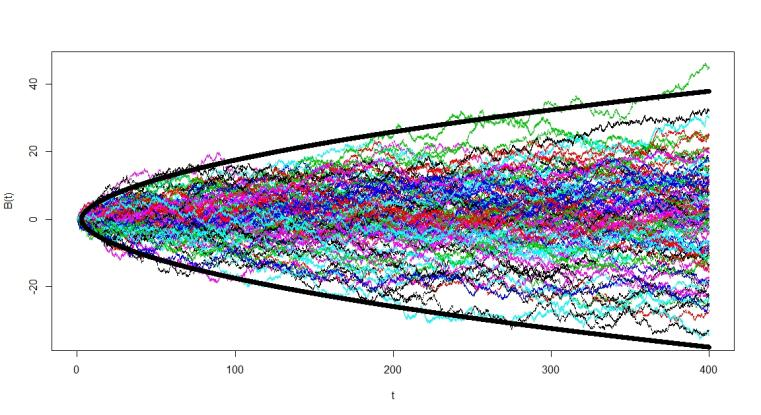### Probability Theory and its applicationsA path simulation of Brownian motion to visualize the iterated logarithm theorem© V. Paulsen / FB 10 / WWU

The focuses of the Institute of Mathematical Stochastics lie in the analysis of complex stochastic systems which appear in numerous fields of natural and social sciences. Amongst others the following topics are of special interest: complex networks, branching processes, percolation theory, renewal theory, statistical mechanics, random matrices, random analytic functions, extreme value theory, large deviations, stochastic approximation, stochastic geometry, optimal transport, financial and actuarial mathematics.

# Prerequisites

A course on Probability Theory that provides a deep knowledge on the following subjects:

Measure and integration, stochastic independence, product spaces, Markov-kernels, 0-1 laws, martingales, laws of large numbers, weak convergence, central limit theorem.

These topics can be found in the book Probability Theory of Prof. Dr. Achim Klenke.

# Courses for the specializations in Probability Theory, its Applications (WA) and Stochastic Processes (SP)

Winter semester 2020/2021

Prof. Dr. Gerold Alsmeyer: Renewal Theory (Type I, II, WA, SP)
Prof. Dr. Steffen Dereich: Stochastic Analysis (Type I, II, WA, SP)
Prof. Dr. Chiranjib Mukherjee: Probability Theory on Trees and Networks (Type I, II, WA, SP)

Summer semester 2021

Prof. Dr. Steffen Dereich: Stochastic Processes (Type, I, II, WA, SP)
Prof. Dr. Zakhar Kabluchko: Concrete Stochastics (Type I,II,WA,SP)
Prof. Dr. Chiranjib Mukherjee: Gaussian free field and Liouville quantum gravity  (Type I,II, WA,SP)
PD Dr. Volkert Paulsen: Advanced Financial Mathematics (Type I, II, WA, SP)

# Further information

The subject areas of the research groups are the following:

Prof. Alsmeyer: renewal theory, branching processes, stochastic fixpoint equations, random discrete structures
Prof. Dereich: complex networks, approximation theory, branching processes, stochastic analysis
Prof. Huesmann: optimal transport, financial mathematics
Prof. Kabluchko: stochastic geometry, randam analytic functions, random discrete structures, extrem value theory
Prof. Löwe: statistical mechanics, random matrices, random graphs, large deviations
Prof. Mukherjee: large deviations, particle systems, stochastic analysis
PD Paulsen: financial and actuarial mathematics

On the sites of the research groups you may find more information about courses, seminars, master-theses, etc.